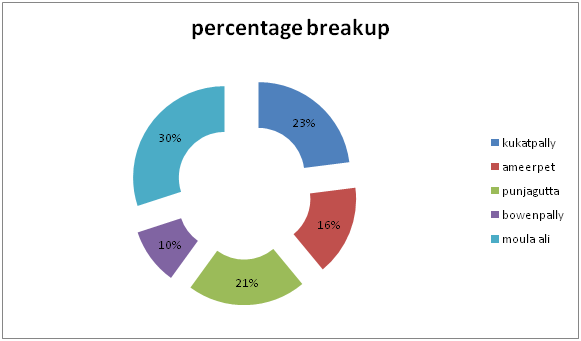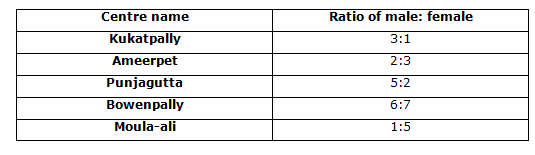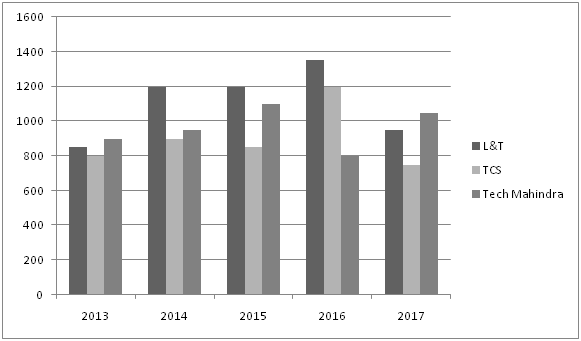# Quantitative Aptitude Questions (Data Interpretation) for RRB Assistant Mains 2018 Day-209

Dear Readers, RRB Assistant is conducting Online Examination for the recruitment of Assistant. To enrich your preparation here we have providing new series of Data Interpretation – Quantitative Aptitude Questions. Candidates those who are appearing in RRB Assistant Mains Exams can practice these Quantitative Aptitude average questions daily and make your preparation effective.

[WpProQuiz 3850]

Click “Start Quiz” to attend these Questions and view Solutions

Directions (1-5): Study the following chart and answer the questions carefully.

Percentage breakup of candidates writing exam in different centers at Hyderabad

Ratio of male to female data given in table

Total students = 3380001) What is the total number of female students have appeared in Bowenpally and Moula-ali centres?

A) 124560

B) 11990

C) 102800

D) 112780

E) 102700

2) If 1/4th of male students have not appeared in examination, then what is the new ratio of male to female in Ameerpet centre?

A) 2:1

B) 1:2

C) 3:1

D) 1:3

E) 5:1

3) Students appeared in Punjagutta centre is how much percent more/less than the Students appeared in Ameerpet branch?

A) 31.25%

B) 41.25%

C) 36.25%

D) 31.35%

E) 26.75%

4) If 25% of students appeared more in the forthcoming year at Kukatpally branch but ratio of male: female is 3:2. What is the total number of female students appeared in forthcoming year?

A) 39876

B) 38870

C) 37780

D) 36570

E) 34567

5) What is the difference between the total number of students appeared in all the centres together and total number of female students appeared in all the centres together?

A) 145677

B) 123147

C) 163137

D) 164147

E) 156432

Directions (Q. 6 – 10) Study the following information carefully and answer the questions given below:

Number of people Recruited by L&T, TCS and tech Mahindra from 2013-2017 are given below:6) For which of the following year the percentage of rise/fall in the recruitment of employees from the previous year is the maximum for the Tech Mahindra?

1. 2015
2. 2016
3. 2017
4. 2014
5. None of these

7) Which company’s average annual recruitment is maximum compared to others?

1. Tech Mahindra and TCS
2. TCS and L&T
3. TCS only
4. L&T only
5. Tech Mahindra Only

8) Total recruitment of TCS in 2014-2016 is approximately what percentage of Total recruitment of L&T in 2015 -2017?

1. 84
2. 91
3. 97
4. 93
5. None of these

9) If 25% of recruited peoples are females in Tech Mahindra means, Find ratio between number of males in Tech Mahindra to total number of employees in L&T.

1. 37:24
2. 24:37
3. 41:37
4. 37:41
5. None of these

10) What is the increase or decrease in the percentage of total number of males in L&T if 10% females are there in every year recruitment, to the number of employees recruited in 2013 -2016 by TCS?

1. 31.7%
2. 35.8%
3. 33.2%
4. 41.2%
5. None of these

Direction (1-5) :

Explanation:

No. of students in Bowenpally = 10% of 338000 =33800

No. of students in Moula-ali = 30%of 338000=101400

Total female students=7/13*33800+5/6*101400

=18200+84500=102700 female students

Explanation:

Total male students appeared in Ameerpet centre is = 338000*16/100

=54080

Male students=54080*2/5

= 21632

1/4th have not appeared (absent for exam) = ¾*21632=16224

Female students = 54080*3/5

=32448
Required ratio = 16224:32448

1:2

Explanation:

Students appeared in Punjagutta = 21/100*338000

=70980

Students appeared in Ameerpet branch = 16/100*338000

=54080

Required percentage = 70980-54080/54080*100

=31.25%

Explanation:

No of students appeared in Kukatpally = 23/100*338000 = 77740

No of students appeared more in the forthcoming year at Kukatpally branch

=125% of 77740

=97175 students

M: F=3:2

2∕5*97175=38870

Explanation:

Total students = 338000

Total female students

= ¼*23/100*338000+3/5*16/100*338000+2/7*21/100*338000+7/13*10/100*338000+5/6*30/100*338000

= ¼*77740+3/5*54080+2/7*70980+7/13*33800+5/6*101400

=19435+32448+20280+18200+84500=174863

Required difference=163137

Direction (6-10) :

Sol:

2014 = 50/950*100 = 5.263 (fall)

2015 = 150/1100 *100 = 13.63 (fall)

2016 = 300 /800 *100 = 37.5 (rise)

2017 = 250/1050 *100 = 23.8 (fall)

2016 rise is maximum.

Sol:

L&T = 5550/5 =1110

TCS = 4500/5 =900

Tech Mahindra = 4800/5 =960

So L&T recruited more avg. employees compared to others

Sol:

Percentage = 2950 /3500 *100 = 84.28 = 84 %

Sol:

Total males in Tech Mahindra = 75/100 * 4800 = 3600

Total number of employees in L&T = 5550

Ratio = 3600:5550 = 24:37

Total number of males in L&T if 10% females

Are there in every year recruitment = 4995

Number of employees recruited in 2013 -2016 by TCS = 3750

Increase in percentage = (4995 -3750) /3750 *100 = 33.2%

Daily Practice Test Schedule | Good Luck

 Topic Daily Publishing Time Daily News Papers & Editorials 8.00 AM Current Affairs Quiz 9.00 AM Quantitative Aptitude “20-20” 11.00 AM Vocabulary (Based on The Hindu) 12.00 PM General Awareness “20-20” 1.00 PM English Language “20-20” 2.00 PM Reasoning Puzzles & Seating 4.00 PM Daily Current Affairs Updates 5.00 PM Data Interpretation / Application Sums (Topic Wise) 6.00 PM Reasoning Ability “20-20” 7.00 PM English Language (New Pattern Questions) 8.00 PM